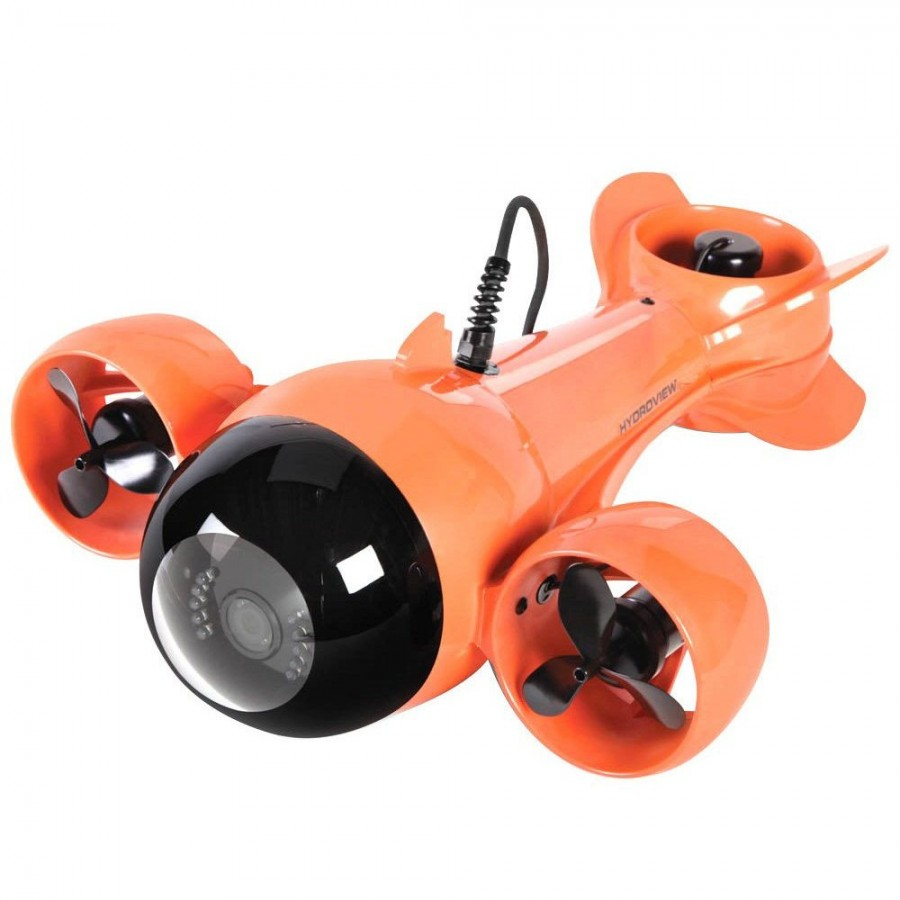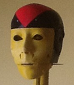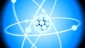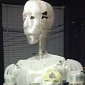# X-Box 360 controller to 3 motors and 2 servos with mrl to run ROV

I am building an underwater drone with a gimbled camera, I thought I had the X-Box controller working last night, but today the same code seems to fail. I see I am using pin6 twice opps

Here is a link to a ROV with the same motor setup:http://www.robotshop.com/en/hydroview-plus-remote-underwater-vehicle.html

The goal is to run the three motors in forward and reverse and run two servos for the camera.

This ran last night, now gives me no data. and any little changes change the available returns fron the joystick. very anoying.

port = "COM4"

servoPin = 6

joystickIndex = 7
servoSpeed = 0.7
servoDirection = 0
servoMin = 0
servoMax = 180

joystick = Runtime.createAndStart("joystick", "Joystick")
arduino = Runtime.createAndStart("arduino", "Arduino")
servoY = Runtime.createAndStart("servoY", "Servo")
servoZ = Runtime.createAndStart("servoZ", "Servo")
rightMotor = Runtime.createAndStart("rightMotor", "Motor")
leftMotor = Runtime.createAndStart("lefttMotor", "Motor")
rearMotor = Runtime.createAndStart("rearMotor", "Motor")

arduino.motorAttach("rightMotor",3,4)
arduino.motorAttach("leftMotor",6,7)
arduino.motorAttach("rearMotor",8,9)

# start the service
joystick = Runtime.start("joystick","Joystick")
print(joystick.getControllers())
python.subscribe("joystick","publishJoystickInput")
joystick.setController(5)

def onJoystickInput(data):
print(data, data.id, data.value)

if data.id == u'6':
print("button 6 is ", data.value)
if data.id == u'z':
print("axis z is ", data.value)

## Comment viewing options### sometimes this works, sometimes it don't, any ideas?

port = "COM4"

joystick = Runtime.createAndStart("joystick", "Joystick")
arduino = Runtime.createAndStart("arduino", "Arduino")

# start the service
joystick = Runtime.start("joystick","Joystick")
print(joystick.getControllers())
python.subscribe("joystick","publishJoystickInput")
joystick.setController(3)

def onJoystickInput(data):
print(data, data.id, data.value)
if data.id == u'6':
print("button 6 is ", data.value)
if data.id == u'z':
print("axis z is ", data.value)### making progress I think

This seems to work 100% of the time as long as I restart mrl every time. It took a while to get rx to work, but now all 5 .data's are captured properly. Now to drive servo's and motors with them.

port = "COM4"

rightMotor = Runtime.createAndStart("rightMotor", "Motor")

joystick = Runtime.createAndStart("joystick", "Joystick")
arduino = Runtime.createAndStart("arduino", "Arduino")

rightMotor.attach(arduino)
rightMotor.setPwmPins(3,4)

# start the service
joystick = Runtime.start("joystick","Joystick")
print(joystick.getControllers())
python.subscribe("joystick","publishJoystickInput")
joystick.setController(3)

def onJoystickInput(data):
print(data, data.id, data.value)
if data.id == u'ry':
print("button ry is ", data.value)
if data.id == u'rx':
print("button rx is ", data.value)
if data.id == u'x':
print("button x is ", data.value)
if data.id == u'y':
print("button y is ", data.value)
if data.id == u'z':
print("axis z is ", data.value)### can not write to a closed port?

Theres a new one. When I connected to motor controller I get an error "can not write to a closed port"

port = "COM4"

rightMotor = Runtime.createAndStart("rightMotor", "Motor")

joystick = Runtime.createAndStart("joystick", "Joystick")
arduino = Runtime.createAndStart("arduino", "Arduino")

rightMotor.attach(arduino)
rightMotor.setPwmPins(3,4)

# start the service
joystick = Runtime.start("joystick","Joystick")
print(joystick.getControllers())
python.subscribe("joystick","publishJoystickInput")
joystick.setController(3)

def onJoystickInput(data):
print(data, data.id, data.value)
if data.id == u'ry':
print("button ry is ", data.value)
if data.id == u'rx':
print("button rx is ", data.value)
if data.id == u'x':
print("button x is ", data.value)
if data.id == u'y':
print("button y is ", data.value)
if data.id == u'z':
print("axis z is ", data.value)
if data.id == 'z':
rightMotor.move(data.value)### You can try to use a code

You can try to use a code similar to this, it worked on 2105 last tested:### thanks, question

what does the abs in: "if (abs(joyX) <= 5):" do?### when i reckon right it

when i reckon right it jchecks if the position of the X-joystick as an absolute value is <= 5, so the direction doesnt matter but only the deflection.

PS: the code definately needs some clean up, i think i messed around with definitions of variables etc### fixed closed com port problem

`arduino ``=` `Runtime.start(``"arduino"``, ``"Arduino"``)`
`arduino.connect(``"COM4"``)`### setPwmPins vs setPwrDirPins / pwm enabled pins

Hi

What type of Arduino are you using ? Not all pins can be used for PWM.

On the Arduino Uno pins 3,5,6,9,10 and 11 can be used.

On the Arduino Mega pins 2-13 and 44-46 can be used.

For other boards please check the reference below

References

https://www.arduino.cc/en/Reference/AnalogWrite

If you use setPwmPins(pinA, PinB) both pins needs to be able to send pwm.

Depending on the motordiriver you use, you can alternativley use

setPwrDirPins(pinA, PinB)

In this case the pinA needs to be a pwm pin, but pinB can be a digital pin.### error

I am using the right pins, when I add Dir to the command I got an error saying service.motorObject has no attribute setPwmDirPins

thank### setPwrDirPins

My misstake

the alternative should be

setPwrDirPins(pinA,pinB)

I don't know why one uses pwm ( like in PulseWidth Modultation ) and the other pwr ( like in Power )

One little letter can make some differnence### no error now but motor still not running

no error now but motor still not running### fixed closed com port problem, fixed setPwrDirPins, still not mo

arduino = Runtime.createAndStart("arduino", "Arduino")
arduino.connect("COM4")

rightMotor = Runtime.createAndStart("rightMotor", "Motor")
leftMotor = Runtime.createAndStart("leftMotor", "Motor")
rearMotor = Runtime.createAndStart("rearMotor", "Motor")

joystick = Runtime.createAndStart("joystick", "Joystick")

rearMotor.setPwrDirPins( 3, 4)
rightMotor.attach(arduino)
leftMotor.attach(arduino)
rearMotor.attach(arduino)
rightMotor.setPwrDirPins( 7, 8)
leftMotor.setPwrDirPins( 5, 6)

# start the service
joystick = Runtime.start("joystick","Joystick")
print(joystick.getControllers())
python.subscribe("joystick","publishJoystickInput")
joystick.setController(3)

def onJoystickInput(data):
print(data, data.id, data.value)
if data.id == u'ry':
print("button ry is ", data.value)
if data.id == u'rx':
print("button rx is ", data.value)
if data.id == u'x':
print("button x is ", data.value)
if data.id == u'y':
print("button y is ", data.value)
if data.id == 'z':
print("axis z is ", data.value)
if data.id == 'z':
rearMotor.move(data.value)### just the basics, motor still does not turn

arduino = Runtime.createAndStart("arduino", "Arduino")
arduino.connect("COM4")

rearMotor = Runtime.createAndStart("rearMotor", "Motor")

rearMotor.setPwrDirPins( 3, 4)
rearMotor.attach(arduino)
rearMotor.move(data.value)### direct arduino control fails, if my code is right.

const int pwm = 3 ;
const int in_1 = 4 ;

void setup() {
// put your setup code here, to run once:
pinMode(pwm,OUTPUT)  ;
pinMode(in_1,OUTPUT) ;

}

void loop() {
// put your main code here, to run repeatedly:
digitalWrite(in_1,HIGH) ;
analogWrite(pwm,255);
delay(3000) ;

}### Example python code to move a motor

The latest build works with the following python to move a motor:

arduino = Runtime.createAndStart("arduino", "Arduino")
arduino.connect("COM7")
rightMotor = Runtime.createAndStart("rightMotor", "Motor")
rightMotor.setPwrDirPins(6,7)
rightMotor.attach(arduino)

rightMotor.move(0.5)

this moves it at 50% speed/power forward.
this works with a dual h-bridge power & direction style motor controller.Bernard McBryan it seems that newer versions of this board have a design flaw, or factory error. Mine did, if you have an unpopulated R7, a populated C20 capacitor, pulldown resistors on the PWM and DIR pins, and P75NF75 mosfets instead of IRF3205, chances are you have this type of faulty board as well. I fixed mine by bridging the R7 connection (I didn't bridge it actually, I ran a wire directly from the 5V input header to the R7-R8 via because I was troubleshooting, but looking at the bottom of the PCB bridging R7 should have the same effect. Try that first, and if the 101 coil gets too hot or whines loudly, do what I did), and you would also need need to turn around the D1 diode. D5 and D3 should have the same orientation, and D1 should be opposite of them. That's it, hopefully that solves your problem as well.﻿

I ordered 2, the one I am testing is indeed missing r&, but the other has it, going to try it now### OK this works!

Had a bad board and while testing it over wrote mrl, so took some time, but this code does work as intended!

The joystick controls both speed and direction of first motor!....

arduino = Runtime.createAndStart("arduino", "Arduino")
arduino.connect("COM4")

rightMotor = Runtime.createAndStart("rightMotor", "Motor")
leftMotor = Runtime.createAndStart("leftMotor", "Motor")
rearMotor = Runtime.createAndStart("rearMotor", "Motor")

joystick = Runtime.createAndStart("joystick", "Joystick")

rearMotor.setPwrDirPins( 3, 4)
rightMotor.setPwrDirPins( 7, 8)
leftMotor.setPwrDirPins( 5, 6)
rightMotor.attach(arduino)
leftMotor.attach(arduino)
rearMotor.attach(arduino)

# start the service
joystick = Runtime.start("joystick","Joystick")
print(joystick.getControllers())
python.subscribe("joystick","publishJoystickInput")
joystick.setController(3)

def onJoystickInput(data):
print(data, data.id, data.value)
if data.id == u'ry':
print("button ry is ", data.value)
if data.id == u'rx':
print("button rx is ", data.value)
if data.id == u'x':
print("button x is ", data.value)
if data.id == u'y':
print("button y is ", data.value)
if data.id == 'z':
print("axis z is ", data.value)
if data.id == 'z':
rearMotor.move(data.value)### OK trying to turn!

arduino = Runtime.createAndStart("arduino", "Arduino")
arduino.connect("COM4")

rightMotor = Runtime.createAndStart("rightMotor", "Motor")
leftMotor = Runtime.createAndStart("leftMotor", "Motor")
rearMotor = Runtime.createAndStart("rearMotor", "Motor")

joystick = Runtime.createAndStart("joystick", "Joystick")

rearMotor.setPwrDirPins( 3, 4)
rightMotor.setPwrDirPins( 7, 8)
leftMotor.setPwrDirPins( 5, 6)
rightMotor.attach(arduino)
leftMotor.attach(arduino)
rearMotor.attach(arduino)

# start the service
joystick = Runtime.start("joystick","Joystick")
print(joystick.getControllers())
python.subscribe("joystick","publishJoystickInput")
joystick.setController(3)

def onJoystickInput(data):
print(data, data.id, data.value)
if data.id == u'x':
savex=x
lefty=y+savex
righty=y-savex
if data.id == u'ry':
print("button ry is ", data.value)
if data.id == u'rx':
print("button rx is ", data.value)
if data.id == u'x':
print("button x is ", data.value)
if data.id == u'y':
#     print("button y is ", data.value)
leftMotor.move(lefty)
rightMotor.move(righty)
if data.id == 'z':
print("axis z is ", data.value)
if data.id == 'z':
rearMotor.move(data.value)### github is for code, not blog posts.

i've moved your code to github , added comments , and fixed the syntax.   I did not test it.. so maybe i had a typo.. but it should give you enough to go on  (hopefully)

You can find the script here:

https://github.com/MyRobotLab/pyrobotlab/blob/master/home/wkinne/rov.py### the link goes to a site that

the link goes to a site that asks for 7000 dollars!!!!! for the thing - I assume you get a seat inside it then and need no joystick????????### yes they are expensive!

thats why I'm building one.### lagg

hey kw, your code to do the x y math causes lagg, a lot of it. Might have to find another way : (

it can get two and three seconds behind.### update frequency

So  I was thnking about it.. the issue is definitley not the "math".. but rather how many updates are being sent to the motors.  Every time any button is pushed in this code, it was updating both the motor speeds.  This was quite ineffecient and as a result, the messages were getting backed up when being sent to the arduino.

the fix is to either downsample the updates to the motor to a rate that the arduino can handle, or some other logic that says only update when you see that the values for x and y have changed....

I checked in a change that will only update the motor speeds when the x or y values were updated..  i didn't test, but i suspect this will do the trick for you..### Thanks!

Yup, that got rid of the lagg, thank you!### I think this all works!

######################################################
# A script to control the ROV
######################################################

# create the arduino service
arduino = Runtime.createAndStart("arduino", "Arduino")
arduino.connect("COM4")

# create services for the 3 motors on the rov
rightMotor = Runtime.createAndStart("rightMotor", "Motor")
leftMotor = Runtime.createAndStart("leftMotor", "Motor")
rearMotor = Runtime.createAndStart("rearMotor", "Motor")
xservo = Runtime.createAndStart("xservo","Servo")
yservo = Runtime.createAndStart("yservo","Servo")

# create the joystick service
joystick = Runtime.createAndStart("joystick", "Joystick")

# set the pins on the motors
rearMotor.setPwrDirPins( 3, 4)
rightMotor.setPwrDirPins( 7, 8)
leftMotor.setPwrDirPins( 5, 6)

xservo.attach(arduino,26)
yservo.attach(arduino,28)

xservo.map(-1,1,0,180)
yservo.map(-1,1,0,180)

# attach the motors to the arduino so they can be controlled
rightMotor.attach(arduino)
leftMotor.attach(arduino)
rearMotor.attach(arduino)

# subscribe to the publishJoystickInput (this will call "onJoystickInput" in python
python.subscribe("joystick","publishJoystickInput")

# choose which controller
joystick.setController(3)

# initialize the values for x and y so they're not null
x = 0
y = 0

################################################################
# This is the joystick callback method
# each button push will call this method with the button id and the value of that button..
################################################################
def onJoystickInput(data):
# define the x and y variables as global
global x
global y
update = False
# a debug statement to print the data being returned from the joystick
print(data, data.id, data.value)
if data.id == u'ry':
print("button ry is ", data.value)
if data.id == 'ry':
yservo.moveTo(data.value)
if data.id == 'rx':
print("button rx is ", data.value)
if data.id == u'rx':
xservo.moveTo(data.value)
if data.id == u'x':
# assign the value to the global variable "x"
x = data.value
update = True
print("button x is ", data.value)
if data.id == u'y':
# assign the joystick value to the global variable y
y = data.value
update = True
# print("button y is ", data.value)
if data.id == 'z':
print("axis z is ", data.value)
if data.id == 'z':
rearMotor.move(data.value)

# at this point we have the latest values for x and y saved in global memory
# compute the values to send to the motors

if update:
lefty=y+x
righty=y-x

# send the values to the motors.

leftMotor.move(lefty)
rightMotor.move(righty)